This is our 9th tutorial of Learning PIC microcontrollers using MPLAB and XC8. Till now, we have covered many basic tutorial like getting started with MPLABX, LED blinking with PIC, Timers in PIC, interfacing LCD, interfacing 7-segment etc. If you are an absolute beginner, then please visit the complete list of PIC tutorials here and start learning.

In this tutorial, we will learn How to Use ADC with our PIC microcontroller PICF877A. Most of the Microcontroller projects will involve an ADC (Analog to Digital converter) in it, because it is one the most used ways to read data from the real world.  Almost all the sensors like temperature sensor, flux sensor, pressure sensor, current sensors, voltage sensors, gyroscopes, accelerometers, distance sensor, and almost every known sensor or transducer produces an analog voltage of 0V to 5V based on the sensors reading. A temperature sensor for instance may give out 2.1V when the temperature is 25C and go upto 4.7 when the temperature is 60C. In order to know the temperature of the real world, the MCU has to just read the output voltage of this temperature sensor and relate it to the real world temperature.  Hence ADC is an important work tool for MCU projects and lets learn how we can use it on our PIC16F877A.

Also check our previous articles on using ADC in other microcontrollers:

### ADC in PIC Microcontroller PIC16F877A:

There are many types of ADC available and each one has its own speed and resolution. The most common types of ADCs are flash, successive approximation, and sigma-delta. The type of ADC used in PIC16F877A is called as the Successive approximation ADC or SAR in short. So let’s learn a bit about SAR ADC before we start using it.

Successive Approximation ADC:  The SAR ADC works with the help of a comparator and some logic conversations. This type of ADC uses a reference voltage (which is variable) and compares the input voltage with the reference voltage using a comparator and difference, which will be a digital output, is saved from the Most significant bit (MSB).  The speed of the comparison depends on the Clock frequency (Fosc) on which the PIC is operating.

Now that we know some basics on ADC, lets open our datasheet and learn how to use the ADC on our PIC16F877A MCU. The PIC we are using has 10-bit 8-channel ADC. This means the output value of our ADC will be 0-1024 (2^10) and there are 8 pins (channels) on our MCU which can read analog voltage. The value 1024 is obtained by 2^10 since our ADC is 10 bit. The eight pins which can read the analog voltage are mentioned in the datasheet. Lets look at the picture below.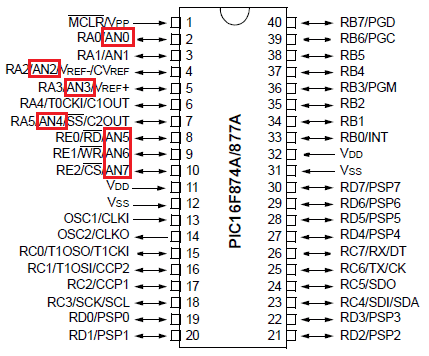The analog channels AN0 to AN7 are highlighted for you. Only these pins will be able to read analog voltage. So before reading an input voltage we have to specify in our code which channel has to be used to read the input voltage.  In this tutorial we will use channel 4 with a potentiometer to read the analog voltage at this channel.

The A/D module has four registers which has to be configured to read data from the Input pins. These registers are:

• A/D Result High Register (ADRESH)

• A/D Result Low Register (ADRESL)

• A/D Control Register 0 (ADCON0)

• A/D Control Register 1 (ADCON1)

The program for using ADC with PIC Microcontroller is very simple, we just have to understand these four registers and then reading any analog voltage will be simple. As usual initialize the configuration bits and let’s start with the void main().

Inside the void main() we have to initialize our ADC by using the ADCON1 register and ADCON0 register. The ADCON0 register has the following bits: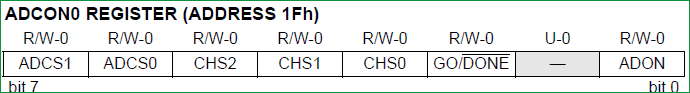In this register we have to turn on the ADC module by making ADON=1 and turn on the A/D Conversion Clock by using the bits ADCS1 and ADCS0 bits, the rest will not be set for now. In our program the A/D conversion clock is selected as Fosc/16 you can try your own frequencies and see how the result changes. Complete details available on datasheet’s page 127. Hence ADCON0 will be initialised as follows.

`ADCON0 = 0b01000001;`

Now the ADCON1 register has the following bits: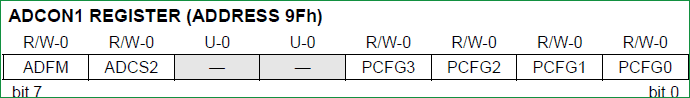In this register we have to make A/D Result Format Select bit high by ADFM=1 and make ADCS2 =1 to select the Fosc/16 again. The other bits remain zero as we have planned to use the internal reference voltage. Complete details available on datasheet page 128. Hence ADCON1 will we set as follows.

`ADCON1 = 0x11000000;`

Now after initializing the ADC module inside our main function, lets get into the while loop and start reading the ADC values. To read an ADC value the following steps has to be followed.

2. Select the analog channel
3. Start ADC by making Go/Done bit high
4. Wait for the Go/DONE bit to get low

1. Initialize the ADC Module: We have already learnt how to initialize an ADC so we just call this below function to initialize the ADC

The void ADC_Initialize() function is be as follows.

```void ADC_Initialize()
{
ADCON1 = 0b11000000; // Internal reference voltage is selected
}```

2. Select the analog channel: Now we have to select which channel we are going to use to read the ADC value. Lets make a function for this, so that it will be easy for us to shift between each channel  inside the while loop.

```unsigned int ADC_Read(unsigned char channel)
{
//****Selecting the channel**///
ADCON0 &= 0x11000101; //Clearing the Channel Selection Bits
ADCON0 |= channel<<3; //Setting the required Bits
//**Channel selection complete***///
}```

Then channel to be selected is received inside the variable channel. In the line

`ADCON0 &= 0x1100101;`

The previous channel selection (if any) is cleared. This is done by using the bitwise and operator “&”. The bits 3, 4 and 5 are forced to be 0 while the others are left to be in their previous values.

Then the desired channel is selected by left shifting the channel number thrice and setting the bits using the bitwise or operator “|”.

`ADCON0 |= channel<<3; //Setting the required Bits`

3. Start ADC by making Go/Done bit high: Once the channel is selected we have to start the ADC conversion simply by making the GO_nDONE bit high:

`GO_nDONE = 1; //Initializes A/D Conversion`

4. Wait for the Go/DONE bit to get low:  The GO/DONE bit will stay high until the ADC conversion has been completed, hence we have to wait till this bit goes low again. This can be done by using a while loop.

`while(GO_nDONE); //Wait for A/D Conversion to complete`

Note: Placing a semi-colon next to while will make the program to be held there till the condtion of the while loop is false.

5. Get the ADC result from ADRESH and ADRESL register: When the Go/DONE bit gets low again it means that the ADC conversion is complete. The result of the ADC will be a 10-bit value. Since our MCU is a 8-bit MCU the result is split into upper 8-bit and the lower 2-bits. The upper 8-bit result is stored in the register ADRESH and the lower 2-bit is stored in the register ADRESL. Hence we have to add up these to registers to get our 10-bit ADC value. This result is returned by the function as shown below:

`return ((ADRESH<<8)+ADRESL); //Returns Result`

The complete function which is used to select the ADC channel, trigger the ADC and return the result is shown here.

```unsigned int ADC_Read(unsigned char channel)
{
ADCON0 &= 0x11000101; //Clearing the Channel Selection Bits
ADCON0 |= channel<<3; //Setting the required Bits
__delay_ms(2); //Acquisition time to charge hold capacitor
GO_nDONE = 1; //Initializes A/D Conversion
while(GO_nDONE); //Wait for A/D Conversion to complete
}```

Now we have a function which will take the channel selection as input and return us the ADC value. Hence we can directly call this function inside our while loop, since we are reading the analog voltage from channel 4 in this tutorial, the function call will be as follows.

`i = (ADC_Read(4)); //store the result of adc in “i”.`

In order to visualize the output of our ADC we will be needing some sort of display modules like the LCD or the 7-segment. In this tutorial we are using a 7-segment display to verify the output. If you want to know how to use 7-segment with pic follow the tutorial here.

The complete code is given below and the process is also explained in the Video at the end.

### Hardware Setup and Testing:

As usual simulate the code using Proteus before actually go with our hardware, the schematics of the project is shown below: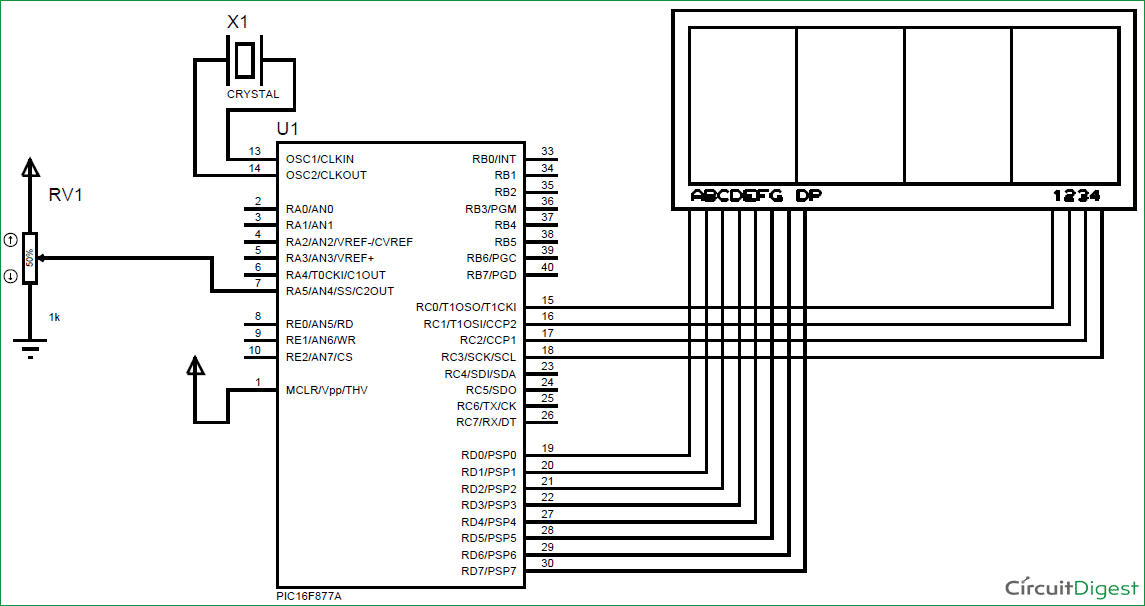Connections of 4-digit seven segment display module with PIC microcontroller are same as the previous project, we have just added a potentiometer to the pin 7 which is the analog channel 4. By varying the pot, a variable voltage will be sent to the MCU which will be read by the ADC module and displayed on the 7-segment display Module. Check the previous tutorial to learn more about 4-digit 7-segment display and its interfacing with PIC MCU.

Here we have used the same PIC Microcontroller board which we have created in LED blinking Tutorial. After ensuring connection upload the program into PIC and you should see an output like this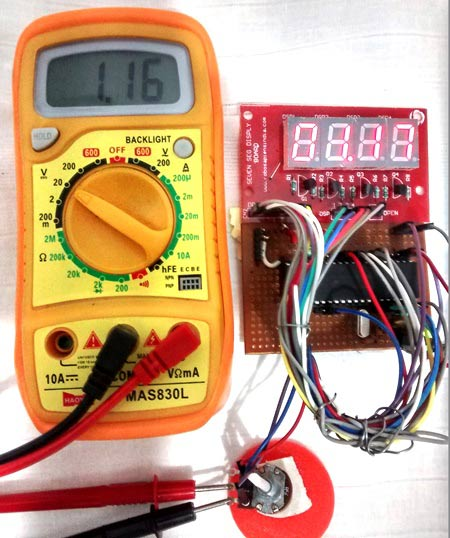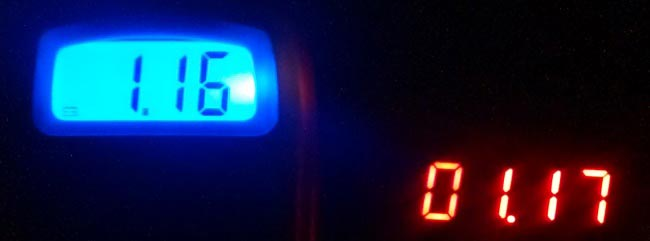Here we have read the ADC value from the pot and converted it to the actual voltage by mapping the 0-1024 output as 0-5 volts (as shown in program). The value is then displayed on the 7-segment and verified using the multimeter.

Thats it, now we are ready to use all the Analog Sensors available in the market, go ahead and try this and if you have any problems as usual use the comment section, we will be happy to help you out.

Code

// CONFIG
#pragma config FOSC = HS        // Oscillator Selection bits (HS oscillator)
#pragma config WDTE = OFF       // Watchdog Timer Enable bit (WDT disabled)
#pragma config PWRTE = ON       // Power-up Timer Enable bit (PWRT enabled)
#pragma config BOREN = ON       // Brown-out Reset Enable bit (BOR enabled)
#pragma config LVP = OFF        // Low-Voltage (Single-Supply) In-Circuit Serial Programming Enable bit (RB3 is digital I/O, HV on MCLR must be used for programming)
#pragma config CPD = OFF        // Data EEPROM Memory Code Protection bit (Data EEPROM code protection off)
#pragma config WRT = OFF        // Flash Program Memory Write Enable bits (Write protection off; all program memory may be written to by EECON control)
#pragma config CP = OFF         // Flash Program Memory Code Protection bit (Code protection off)

// #pragma config statements should precede project file includes.
// Use project enums instead of #define for ON and OFF.

#include <xc.h>
#define _XTAL_FREQ 20000000

//***Define the signal pins of all four displays***//
#define s1 RC0
#define s2 RC1
#define s3 RC2
#define s4 RC3
//***End of definition**////

{
ADCON1 = 0b11000000; // Internal reference voltage is selected
}

{
ADCON0 &= 0x11000101; //Clearing the Channel Selection Bits
ADCON0 |= channel<<3; //Setting the required Bits
__delay_ms(2); //Acquisition time to charge hold capacitor
GO_nDONE = 1; //Initializes A/D Conversion
while(GO_nDONE); //Wait for A/D Conversion to complete
}

void main()
int i = 0; //the 4-digit value that is to be displayed
int flag =0; //for creating delay

unsigned int seg[]={0X3F, //Hex value to display the number 0
0X06, //Hex value to display the number 1
0X5B, //Hex value to display the number 2
0X4F, //Hex value to display the number 3
0X66, //Hex value to display the number 4
0X6D, //Hex value to display the number 5
0X7C, //Hex value to display the number 6
0X07, //Hex value to display the number 7
0X7F, //Hex value to display the number 8
0X6F  //Hex value to display the number 9
}; //End of Array for displaying numbers from 0 to 9

//*****I/O Configuration****//
TRISC=0X00;
PORTC=0X00;
TRISD=0x00;
PORTD=0X00;
//***End of I/O configuration**///

#define _XTAL_FREQ 20000000

while(1)
{

if(flag>=50) //wait till flag reaches 100
{
flag=0; //only if flag is hundred "i" will get the ADC value
}
flag++; //increment flag for each flash

//***Splitting "i" into four digits***//
a=i%10;//4th digit is saved here
b=i/10;
c=b%10;//3rd digit is saved here
d=b/10;
e=d%10; //2nd digit is saved here
f=d/10;
g=f%10; //1st digit is saved here
h=f/10;
//***End of splitting***//

PORTD=seg[g];s1=1; //Turn ON display 1 and print 4th digit
__delay_ms(5);s1=0;     //Turn OFF display 1 after 5ms delay
PORTD=seg[e];RD7=1;s2=1; //Turn ON display 2 and print 3rd digit
__delay_ms(5);s2=0;     //Turn OFF display 2 after 5ms delay
PORTD=seg[c];s3=1; //Turn ON display 3 and print 2nd digit
__delay_ms(5);s3=0;     //Turn OFF display 3 after 5ms delay
PORTD=seg[a];s4=1; //Turn ON display 4 and print 1st digit
__delay_ms(5);s4=0;     //Turn OFF display 4 after 5ms delay

}
}

Video

Subscribe below to receive most popular news, articles and DIY projects from Circuit Digest

•Pankaj
Apr 16, 2017

Please tell why we have to shift channel 3times

• Aswinth Raj
Apr 17, 2017

Hi Pankaj,

To understand why I have shifted the bits thrice, you hav to look at the ADCON0 register in he datasheet. It will be shown at page 127 of the PIC16F877A datasheet.

As you can see the CHS0,CHS1 and CHS2 are used to set the required channel for ADC. They are positioned after three bits from the right, hence in order to access them we left shift our values thrice and update the channel by using the or "|" operator.

•Dayanand Killedar
Apr 28, 2017

And please explain me the Right Justification & Left Justification.
Thank u.

• Aswinth Raj
Apr 28, 2017

The operator "<<" is called Left Shifting operator. You have to google to know more about it.

The explanation of the Line is given below

The result of the ADC will be a 10-bit value. Since our MCU is a 8-bit MCU the result is split into upper 8-bit and the lower 2-bits. The upper 8-bit result is stored in the register ADRESH and the lower 2-bit is stored in the register ADRESL. Hence we have to add up these to registers to get our 10-bit ADC value. This result is returned by the function as shown below:

`return ((ADRESH<<8)+ADRESL); //Returns Result`

•Abel
Jun 29, 2017

please from '' i = adc*0.488281; '' where is 0.488281 from? what calculation did you do that gave you that?
and also please give me an example of the original value that will be stored in *adc* or the ADC_Read() function because i would love to know the original value of 'i' at each increment and decrements.

• Aswinth Raj
Jun 29, 2017

Hi Abel,

Here, the variable adc will have the ADC value which was returned by the function ADC_Read(). This value will be 0 for 0V and 1024 for 5V.

Now I have to display the voltage on a 7-segment display. So the value stored in adc should be converted to actual voltage level that can displayed. hence I multiply the adc value with 0.488281 so that I could convert it into actual voltage.

For Example: Lets say my Input voltage is 5V, the PIC will read it and store it as 1024 in my "adc" variable. When that is multiplied with 0.488281 it gives 499 (decimal neglected since "i" is an integer) now I can directly display this 499 on the 7-segment display just by adding a point before two digits.

Generally the value "0.488281" is called as the multiplication factor and would vary based on your application.

HAPPY LEARNING!!

Thanks

•Abel
Jul 14, 2017

wow!! thanks a lot, you cleared my confusion..
please what if i want it to display from 5-0v on LCD... what would be the maths.. instead of the adc*0.488128.. i tried (voltage= (adc*5)/1024 ; but not getting the result

• Aswinth Raj
Jul 14, 2017

Should have worked. Make sure the variable voltage is in float type. If still faing problems simply

multiply it with 0.0048128 to convert 0-1024 into 0-5

•Abel
Jul 15, 2017

Thanks so much... The variable wasn't in float type that was what caused the problem ... thanks

•Abel
Jul 15, 2017

Thanks for your relies so far... sorry from ADCON0 |= chennel<<3.. what if i wish to use other channel, like channel 3, 5, 6 and 7??

• Aswinth Raj
Jul 15, 2017

Hi Abel,

Remains the same for all ADC channels.

In the example program above the line

Please, use the forum to post your questions. So that everyone can be benifited out of it.

Thanks,...

•Sep 12, 2017

I am using CCS C compiler.Can you give me the C programme for pic 16f886

•Felix Noussi
Jul 18, 2017

bonjour Monsieur et merci pour vos tuto
s'il vous plait j'ai besoin d'aide pour l'ADC du 18f4431 .

•salman
Aug 06, 2017

sir
i m not understand why u used flag

•salman
Aug 08, 2017

sir how you show decimal point in seven segment display. because u not declare decimal point in seg[]

• Mujtaba
Oct 09, 2020

PORTD=seg[e];RD7=1;s2=1; //Turn ON display 2 and print 3rd digit........ Here RD7 is for decimal

•Sep 11, 2017

To compile this programme in CCS 'C' compiler! What are the changes required

•Phong Tran
Oct 12, 2017

Hi Raj,
I'm new to coding and ran across your post but don't know how to apply to my project. See if you can shed some light.
2 Digits display
Port E is line date (digit 1,2)
PortD is Segment
Pot (ADC) is on Channel A0, and I use MCC to configure the Ports

Thanks you

•Abdul
Nov 06, 2017

Hi,
Well explained article.
I am taking variable input range 85 to 45 VDC by using voltage divider.
1 - How much input Voltage I can take on AN0 (say 10V max or only 5v max)?
2 - What could be best formula to take 0.01V resolution to be used for PWM output for above input voltage?

thank you
would be better if you reply by email as well.

• AISHA
Nov 14, 2017

The maximum input voltage of the ADC is 5V. The resolution depends on the number of bit

•Abd Rafiu
Nov 27, 2017

Good day. Honestly speaking, I have been kept silent for over three hours, reading your articles. Very explicit and unambiguous straight forward articles. I really appreciate your efforts and time you created for the whole explaination. may God Almighty Allah continue to increase your knowledge of understanding. Now my question is that, assume I want to configure two or more different input analog such as RA0(AN0), and RA1(AN1) to have independent input e.g sensor and other supply voltage to be displayed on LCD etc, how I am going to arrange the function . Please explain it to me the way you have cleared the article above.

•Inzunza
Feb 25, 2018

Hello
that such a friend, I would like to know if I could pass the simulation of the file because I try to simulate the diagram and not only appear zeros and I move the potentiometer and I keep appearing the same

• Aswinth Raj
Feb 26, 2018

I dont think I still have my simulation file with me. Why dont you share your screenshot of the simulation. I can tell you where you have made the mistake

• venkatesh
Feb 19, 2021

Hello,

I am new to the program. I need to understand the program which you had done. I have simply fed your program to the PIC and seeing nothing. Also I think you had done a mistake in that mapping. Its actually 0.00488*adc. In program it is 0.488*adc. Please clarify

Thanks

• mojtaba
Jul 18, 2021

Dear venkatesh, yes it is actually 0.00488** but in the 7 segment we want to display 2 digit after decimal point. since int type variable in C takes only integer( 0 , 1, 2..) and delete whatever after decimal point so you should multiply by 100 to get two digit after decimal point percision. if you look at line 92 RD7=0; meand decimal point then 2 digit. so multipication factor is 0.00488**x100 = 0.488**

• mojtaba
Jul 18, 2021

i had same problem like you in simulation but i figured out that 7 segment is common cathod and the port S0, S1,S2 and S3 must be LOW to display the digit , and must be high to turn of the 7segment unit .

so in line 90 until 97 of program i swith Sn=0 and Sn=1 . after that my program worked fine

• rehpej
Sep 26, 2018

Good day,

I am using PIC18F4685 and trying to understand ADC with this microcontroller (I just started a week ago with microcontroller). And I'm confused with the ADCON registers.

ADCON1 = 0b00000000; // Bit5 as AVss Bit4 as AVdd
ADCON2 = 0b10???001; /* Bit7 as Right Justified
Bits5-3 I don't know how to select the TAD
Bits2-0 Fosc/8, I am using __XTAL_FREQ 8000000 (Please do correct me)*/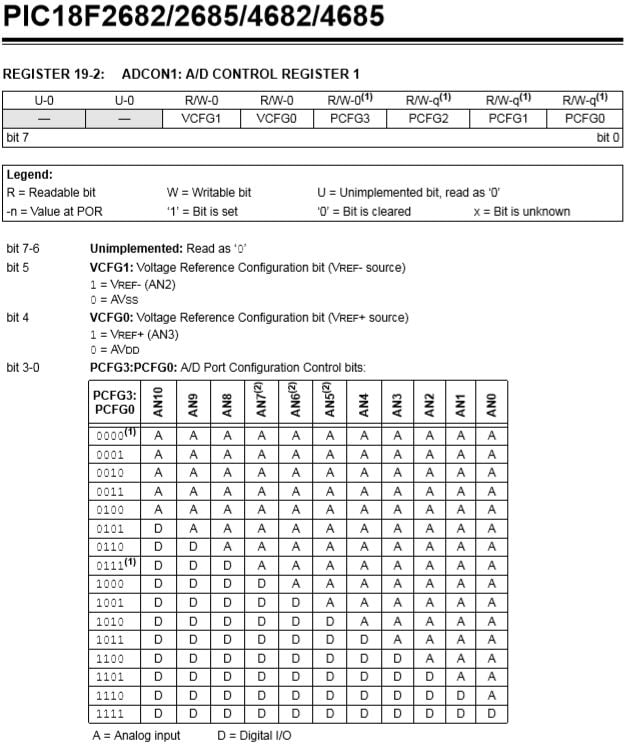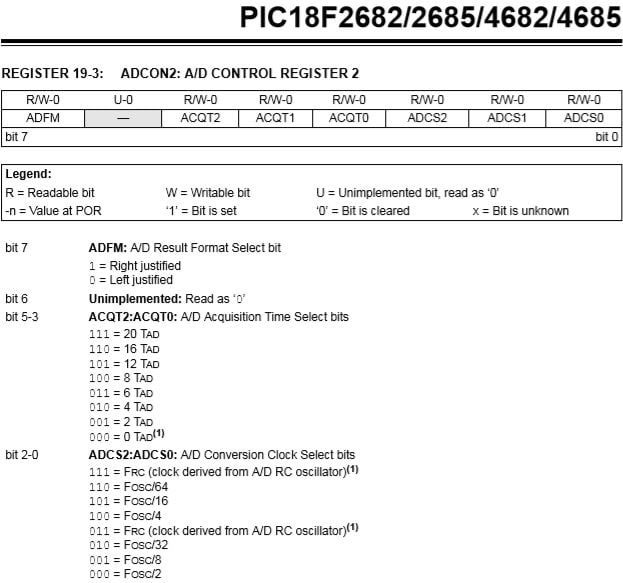How do I choose the ACQT bits? Can I set them to 000 or 0TAD as for now?

I am using a Hall Effect Module as my analog input, a PIC18F4685, MPLAB XC8 as my compiler, PICkit3 as my programmer.

Thanks

• rehpej
Sep 26, 2018

Good day,

I'm new to PIC programming, and I'm lost on how to set the ADCON registers for my PIC. I am using PIC18F4685 and it have ADCON0, 1 and 2. I have set my ADCON base on the PIC datasheet.

ADCON1 = 0b00000000; // Bit5 as AVss Bit4 as AVdd
ADCON2 = 0b10101001; /* Bit7 as Right Justified
Bits5-3 I don't know how to select the TAD
Bits2-0 Fosc/8, I am using __XTAL_FREQ 8000000 */

At ADCON0, I have turned on the ADC and select channel0 (RA0). But what if I want to select other channels since my PIC have 8 analog channels.

At ADCON1, reference voltage is the supplied voltage (bits 5 and 4). And the port conf bits are all analog input (bits 3 to 0). Bits 7 and 6 are unimplemented.

At ADCON2, this part is where I am confused. Bit 7 is right justified and Bit 6 is unimplemented.

Bits 5 to 3 is set to 101 or 12TAD, I have search to google and the minimum TAD for a 10bit ADC is 12TAD. How to know what TAD to choose? At the datasheet, A/D clock period minimum is at 0.7us and maximum is at 25us.

Bits 2 to 0 is set to 101 or FOSC/16. Can I choose anything? I am using a crystal oscillator at 8MHz.

Please do correct me if I'm wrong. I am still new to this and need some guidance.

Thank you.

• Joseph Tannenbaum
Jun 20, 2020

I'm using a common anode display, so the seg's have to be complimented.  I don't see how you get the decimal point.  Mine is reading fine, but no decimal point.

• Joseph Tannenbaum
Jun 20, 2020

Sorry, never mind, I found where you turn on RD7..Had to make it =0 to turn on the decimal point.

• CV
Apr 09, 2021

Thank you, this was very helpful.
But please note there is at least two places in the sample code where 0x... should be 0b...

```ADCON1 = 0x11000000;  <---should be 0b11000000

ADCON0 &= 0x11000101; //Clearing the Channel Selection Bits  <-- should be 0b11000101

```

I did a cut and paste and it took me a while to figure out why things weren't working!

• mojtaba
Jul 18, 2021

Hi dear Aswinth Raj, in the funtion below